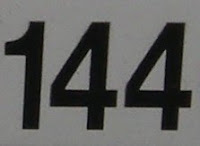## Thursday, June 3, 2010

### 144

144 = 24 x 32.

1442 = 122, a perfect square. If you reverse the digits, 212 = 441.

144 has a unique representation as a sum of three squares: 144 = 42 + 82 + 82.

144 is a square number. Divide it by 4 and you get 36, which is also square. Does this happen with any other square numbers?

144 is the 12th Fibonacci number.

144 is 99 in base 15.The interior angles in a regular decagon measure 144 degrees. In counting objects, 144 is called a gross (a dozen dozens).

Source: WolframAlpha

#### 1 comment:John C. George said...

You say, "144 is a square number. Divide it by 4 and you get 36, which is also square. Does this happen with any other square numbers?"

The question is ambiguous. Clearly 100, for instance, is a square which, when divided by 4 gives you 25 which is also a square. So indeed any even square must have the property that dividing it by 4 gives you another square. To what property does the word "this" in the question refer?